/

### Annualisation Conventions: 1. Introduction

Next section

A statement from a bank or insurer that a deposit will be credited with interest at the rate of 10% per annum may sound unambiguous. In fact it is not. Interest rates and yields (and hence spreads between different interest rates) can be quoted in a variety of ways.

First we note that market interest rate levels will differ according to the term of the deposit. Thus in practice we might find that the interest rate credited on 1 year deposits is 5% per annum, but on 2 year deposits is 6% per annum, say. This dependency on time is called the ‘yield curve’. Interest bearing bonds will have a ‘running’ (or ‘coupon’) yield, which is the interest per unit nominal received each year. However, as their price may not be at par, attention is more usually focused on their ‘redemption yield’, i.e. the rate of return needed to equate their current value with the present value of all future payments, both income and redemption proceeds. With equities, which pay uncertain levels of dividends, the ‘dividend yield’ may refer to the current dividend rate divided by the current price. The ‘rental yield’ is a corresponding term that is used for property, i.e. real estate, referring here to the rental income that the property might provide. All of the above may be quoted gross or net of tax if relevant (and potentially also gross or net of other types of expenses).

However these various definitions of yield still do not exhaust the range of meanings that can be placed on a quoted interest rate. In particular, the meaning to be ascribed to any particular interest rate depends on the ‘annualisation convention’ being adopted.

If, say, a 10% per annum interest rate is being quoted with annual compounding the statement that  the interest rate is 10% per annum means that at the end of one year 100 grows to: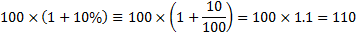To be meaningful we of course also need to specify the currency (or more generally the ‘numeraire’) in which value is being expressed, e.g. US\$, GB£, Euro, Yen, ....

However, when the interest rate is expressed with semi-annual compounding, then the statement means that we earn 5% every six months. This means that at the end of one year 100 grows to: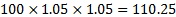When the interest rate is expressed with quarterly compounding, then the statement means that we earn 2.5% every 3 months. This means that at the end of one year 100 grows to: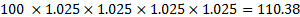We can generalise these results by supposing that an amount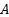is invested foryears at an interest rate ofthat is compounded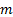times per annum. The terminal value of the investment is then: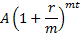The limit as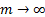is called continuous compounding. The terminal value is then: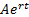Here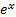, also called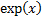or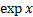, is the exponential function, i.e.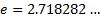raised to the power of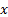. The continuously compounded interest rate (sometimes also called the force of interest) equivalent to a 10% annually compounded interest rate is thus: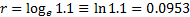The difference between interest rates quoted with different annualisation conventions increases the further the interest rate deviates from 0%, as is illustrated in Table 1. For most practical purposes, continuous compounding can be thought of as equivalent to daily compounding.